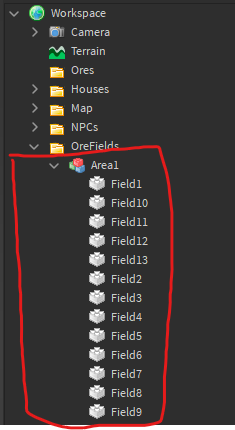# Raycasts creates rays outside predefined field

Hi, so I have this group of partsEach part is a basic rectangle part meant to create this group above my map

For whatever reason, rays keep spawning outside the fields

First of all, im using a function to pick a random field based on the part’s mass so small fields can’t spawn as many rays as big fields

``````local function pick_field_from_model()
local fields = {}

for i, v in pairs(game.Workspace.OreFields[area]:GetChildren()) do
local mass = math.floor(v.Mass / 10)
for i = 1, mass do
table.insert(fields, v)
end
end

local chosen_field = fields[math.random(1, #fields)]
return chosen_field
end
``````

The next part is the main raycast function

``````local function spawn_ore()
local chosen_field = pick_field_from_model()

local x = chosen_field.Position.X
local z = chosen_field.Position.Z

local xSize = chosen_field.Size.X/2
local zSize = chosen_field.Size.Z/2

local pos1 = math.random(x-xSize, x+xSize)
local pos2 = math.random(z-zSize, z+zSize)

local rayOrigin = Vector3.new(pos1, chosen_field.Position.Y, pos2)
local rayDirection = Vector3.new(0,-500, 0)
local raycastParams = RaycastParams.new()
raycastParams.FilterType = Enum.RaycastFilterType.Whitelist
raycastParams.FilterDescendantsInstances = {workspace.HexMapBin.HexMap.Cells}

local raycastResult = workspace:Raycast(rayOrigin, rayDirection, raycastParams)
if raycastResult then
local hit = raycastResult.Instance

if hit.Color == Color3.fromRGB(R, G, B) then

local distance = (rayOrigin - hit.Position).Magnitude
local p = Instance.new("Part")
p.Anchored = true
p.CanCollide = false
p.Parent = workspace.Ores
p.Size = Vector3.new(0.1, 0.1, distance)
p.CFrame = CFrame.lookAt(rayOrigin, hit.position)*CFrame.new(0, 0, -distance/2)
game.ServerStorage.OreFields[area].Value += 1
end
end
end
``````

Anyone knows why the rays spawn outside the fields?

Ok figured this out. The new raycast requires you to also add the orientation if the part’s orientation is not 0,0,0.
`local rayOrigin = (chosen_field.CFrame * CFrame.new(pos1, 0, pos2)).p`